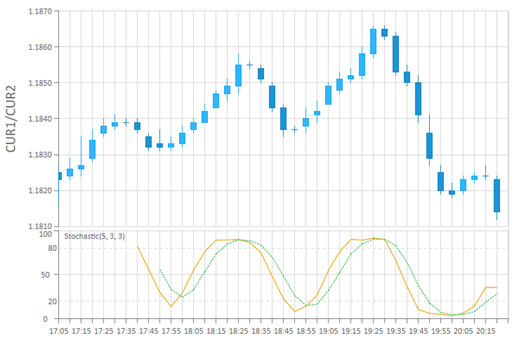BCGControlBar Pro for MFC
CBCGPChartStochasticFormula

## Detailed DescriptionA Stochastic indicator is represented by two lines. The main line is called %K, the second - %D, which is a moving average (MA) of %K. The formula itself implements %K. To display %D just create CBCGPChartMAFormula with desired MA type using the output series of %K as an input series.

The following parameters should be defined for Stochastic:

• Period of KSlowing of %K, which defines the internal smoothing of %K line.
• %D - period of MA applied to %K and a type (simple, exponential, snooted, linear weighted) of MA.

The following example demonstrates how to set up a Stochastic Oscillator with periods 5, 3, 3 and display it on a custom axis (making up an indicator area):

Copy
CBCGPChartAxis* pAxisStochastic = (CBCGPChartAxisY*) pYAxis->Split(80, 3, NULL, FALSE);
pAxisStochastic->SetFixedDisplayRange(0, 100, 50);
CBCGPChartStockSeries* pStockSeries = new CBCGPChartStockSeries(pChart, CBCGPBaseChartStockSeries::SST_CANDLE);
CBCGPChartStochasticFormula stochastic(5, 3);
CBCGPChartSeries* pStochSeries = stochastic.Create(pChart, _T("Stochastic"), pStockSeries, pAxisStochastic);
CBCGPChartMAFormula signalStoch(CBCGPChartMAFormula::MA_SIMPLE, 3);
CBCGPChartSeries* pSignalStochSeries = signalStoch.Create(pChart, _T("Stochastic Signal"), pStochSeries, pAxisStochastic);

If you provide a main stock series as an input series, the formula implementation will automatically take high, low and close child series to compute the output series.Inheritance diagram for CBCGPChartStochasticFormula:

## Public Member Functions

GetKPeriodGets K period.

GetSlowingGets slowing of %K.Public Member Functions inherited from CBCGPChartAdvancedFormula
CreateCreates a formula.

GeneratePointsCalled to generate data points for an output series.

GetOutputSeriesCategoryReturns output series category.

GetOutputSeriesTypeReturns output series type.

GetPeriodReturns formula period.

SetOutputSeriesCategorySets output series category.

SetOutputSeriesTypeSets output series type.

SetPeriodSets formula period.Public Member Functions inherited from CBCGPChartBaseFormula
FindInputSeriesIndexFinds input series index.

GetInputSeriesAtReturns an input series located at the specified position.

GetInputSeriesCountReturns the number of input series.

GetLParamReturns user-defined data.

GetParentSeriesReturns a pointer to a parent series.

IsNonDiscreteCurveTells whether the formula is displayed as a non-discrete curve.

SetLParamSets user-defined data.

SetParentSeriesSets a parent series that represents a formula on the diagram.Public Attributes inherited from CBCGPChartAdvancedFormula